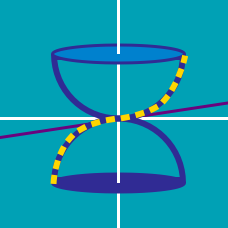Calculus

# Volume of Revolution: Level 4 Challenges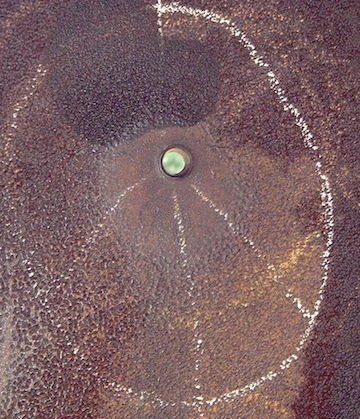A bullet is formed by revolving the area bounded by the the curve $y = \ln(x)$ from $x = 1$ to $x = e$ about the $x$-axis.

It is then shot straight into a very thick wall (i.e. it does not pierce through the other side at all) making a closed cylindrical hole until it stops moving. Then the bullet is carefully extracted without affecting the hole at all, leaving an empty hole with a pointy end where the bullet once was.

The length of the entire hole is $e+1$. If the volume of the hole can be expressed as $\pi i e,$ where $i$ is a constant, find the value of $i$.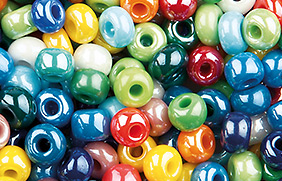The beads for a bracelet are spheres of radius of 5mm with a hole of radius 3mm drilled through the center so that they can be threaded together.

What is the volume, in mm$^3$, of material removed from the bead when the hole is drilled?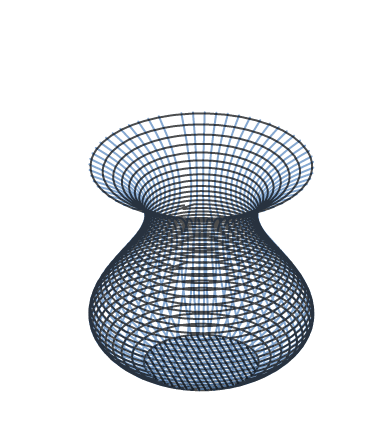A vase maker decides to construct a vase whose contour is a cubic polynomial according to the following specifications:

$\bullet$ The vase is 4 feet tall.
$\bullet$ The vase is 4 feet in diameter at its widest (which occurs 1 foot from the base).
$\bullet$ The vase is 2 feet in diameter at its narrowest (which occurs 1 foot from the top).
$\bullet$ The vase has a flat circular bottom.

Rounded to 3 decimal places, what is the volume of the vase in cubic feet?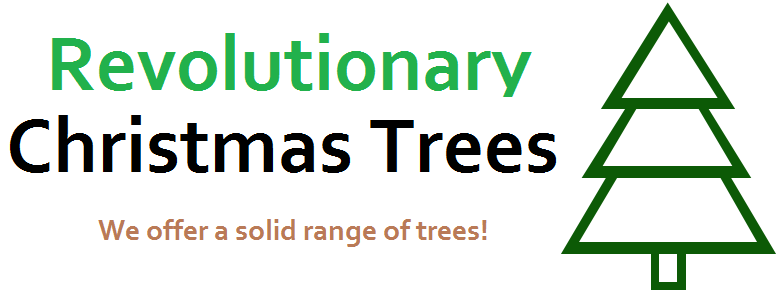A mathematician is buying a Christmas tree from Revolutionary Christmas Trees.

He sends this model function ( $x$ in terms of $y$ ):

$x = \begin{cases} 2 - ( \frac{\lfloor y \rfloor }{2} + \{ y \} ) & 0 \leq y < 3\\ \frac{1}{2} & -1 \leq y < 0\\ 0 & \text{otherwise} \end{cases}$

This is rotated round the $y$-axis to create a solid of revolution to model his tree.

He then sends a volume enlargement scale factor $s$ by which the volume of the solid is multiplied to make the tree the correct size. He wishes to have a final volume of $640 \pi$.

Find the value of $s$.

Note: The notation {$y$} means the fractional part of $y$

The volume of the solid formed by revolving the curve

$y= \frac{1}{\sqrt{x}} - \frac{1}{\sqrt{x+1}}$

bounded between $x = \frac{1}{8}$ and $x=\frac{9}{16}$ around the $x$-axis is in the the form $A \pi \ln{\frac{B}{C}}.$

If $A$ and $B$ are square-free, what is the value of $A \times B \times C?$

×